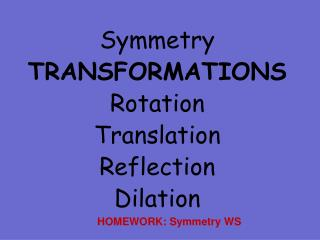Download PresentationSymmetry TRANSFORMATIONS Rotation Translation Reflection Dilation

# Symmetry TRANSFORMATIONS Rotation Translation Reflection Dilation - PowerPoint PPT Presentation

Symmetry TRANSFORMATIONS Rotation Translation Reflection Dilation. HOMEWORK: Symmetry WS. Symmetry. What is a line of symmetry?. A line on which a figure can be folded so that both sides match. To coincide. To be a line of symmetry, the shape must have two halves that match exactly.I am the owner, or an agent authorized to act on behalf of the owner, of the copyrighted work described.
Download Presentation## Symmetry TRANSFORMATIONS Rotation Translation Reflection Dilation

Download Policy: Content on the Website is provided to you AS IS for your information and personal use and may not be sold / licensed / shared on other websites without getting consent from its author.While downloading, if for some reason you are not able to download a presentation, the publisher may have deleted the file from their server.

- - - - - - - - - - - - - - - - - - - - - - - - - - E N D - - - - - - - - - - - - - - - - - - - - - - - - - -
Presentation Transcript
1. Symmetry TRANSFORMATIONS Rotation Translation Reflection Dilation HOMEWORK: Symmetry WS

2. Symmetry

3. What is a line of symmetry? • A line on which a figure can be folded so that both sides match. To coincide.

4. To be a line of symmetry, the shape must have two halves that match exactly. When you trace half a heart onto a piece of folded paper, and then cut it out, the two half hearts make a whole heart. The two halves are symmetrical.

5. United States of America Canada Maryland England Which of these flags have a line of symmetry? No No

6. - What about these math symbols? Do they have symmetry? + - Yes Yes x Yes Yes

7. How many lines of symmetry to these regular polygons have? 5 4 3 Do you see a pattern? 8 6

8. Identify the letters that are Horizontally or Vertical Lines of Symmetry A B C D E FG H I J K L M N O P Q R S T U V W X Y Z Infinite number

9. Symmetrical Words A H I M O T U V W X Y Vertically Symmetric B C D E H I K O X Horizontally Symmetric

10. Rotational Symmetry(Turn) The action of turning a figure around a point or a vertex.

11. Rotational Symmetry

12. Rotational Symmetry all around us Click to see the answers. Which road signs have got rotational symmetry?

13. Rotational Symmetry all around us Which road signs have got rotational symmetry?

14. Identify the Rotationally Symmetric Letters A B C D E FG H I J K L M N O P Q R S T U V W X Y Z

15. Maryland Letters may possess rotational symmetry. H      I      N      O      S      X      Z H      I      N      O      S      X      Z Flags may possess rotational symmetry.

16. Point Symmetry A figure also has point symmetry if it can be rotated 180 degrees. 360/4 = 90 two rotation make 180

17. Examples: Name the type(s) of symmetry each figure has. reflection Rotation of 120 Rotation and point reflection

18. Click the triangle to see rotation Turning a figure around a point or a vertex Rotation

19. Translation (Slide) The action of sliding a figure in any direction.

20. Click the Octagon to see Translation. Translation The act of sliding a figure in any direction.

21. Reflection (flip) The result of a figure flipped over a line.

22. Reflection Click on this trapezoid to see reflection. The result of a figure flipped over a line.

23. Which shapes show reflection, translation, or rotation? Reflection Rotation Translation